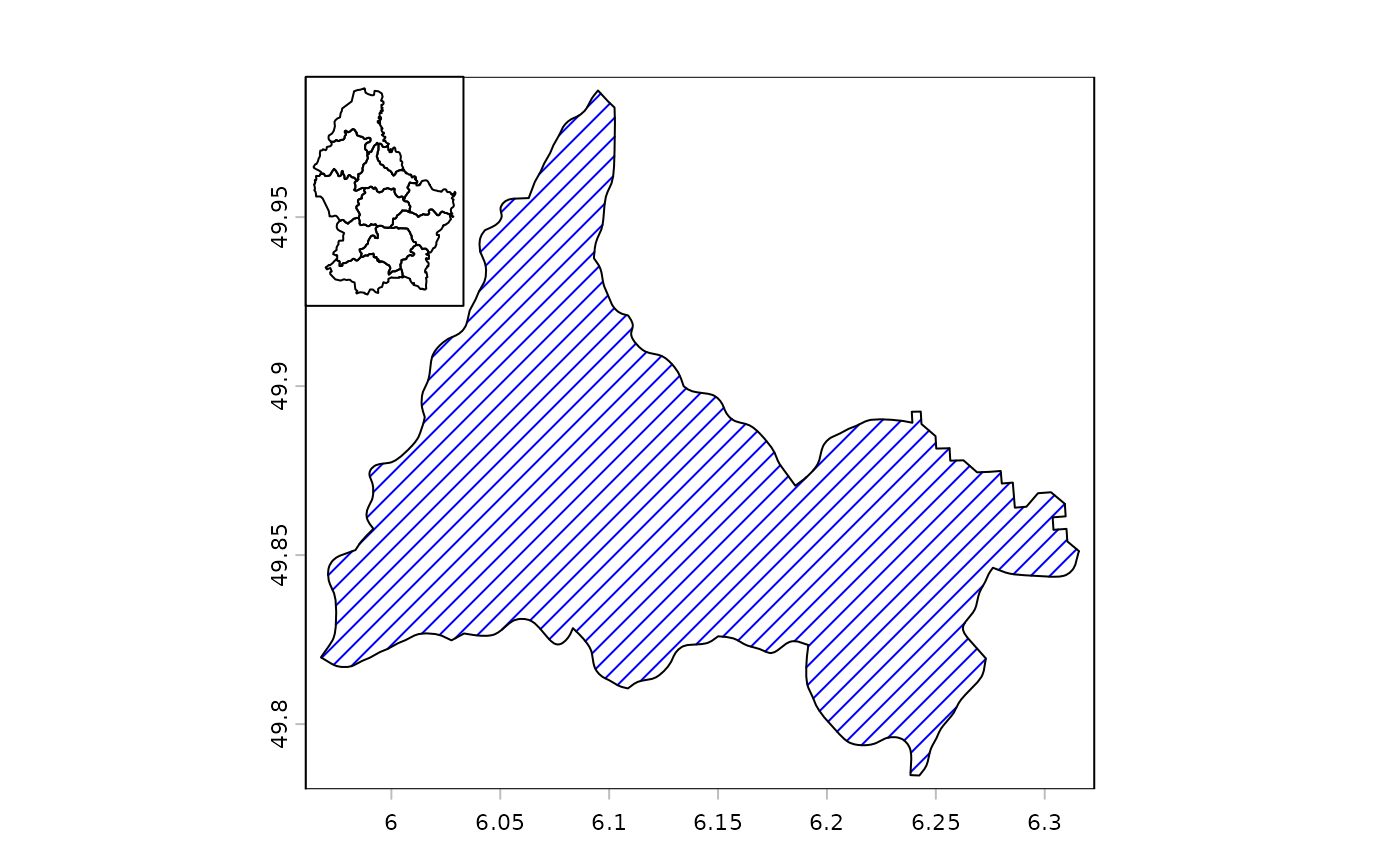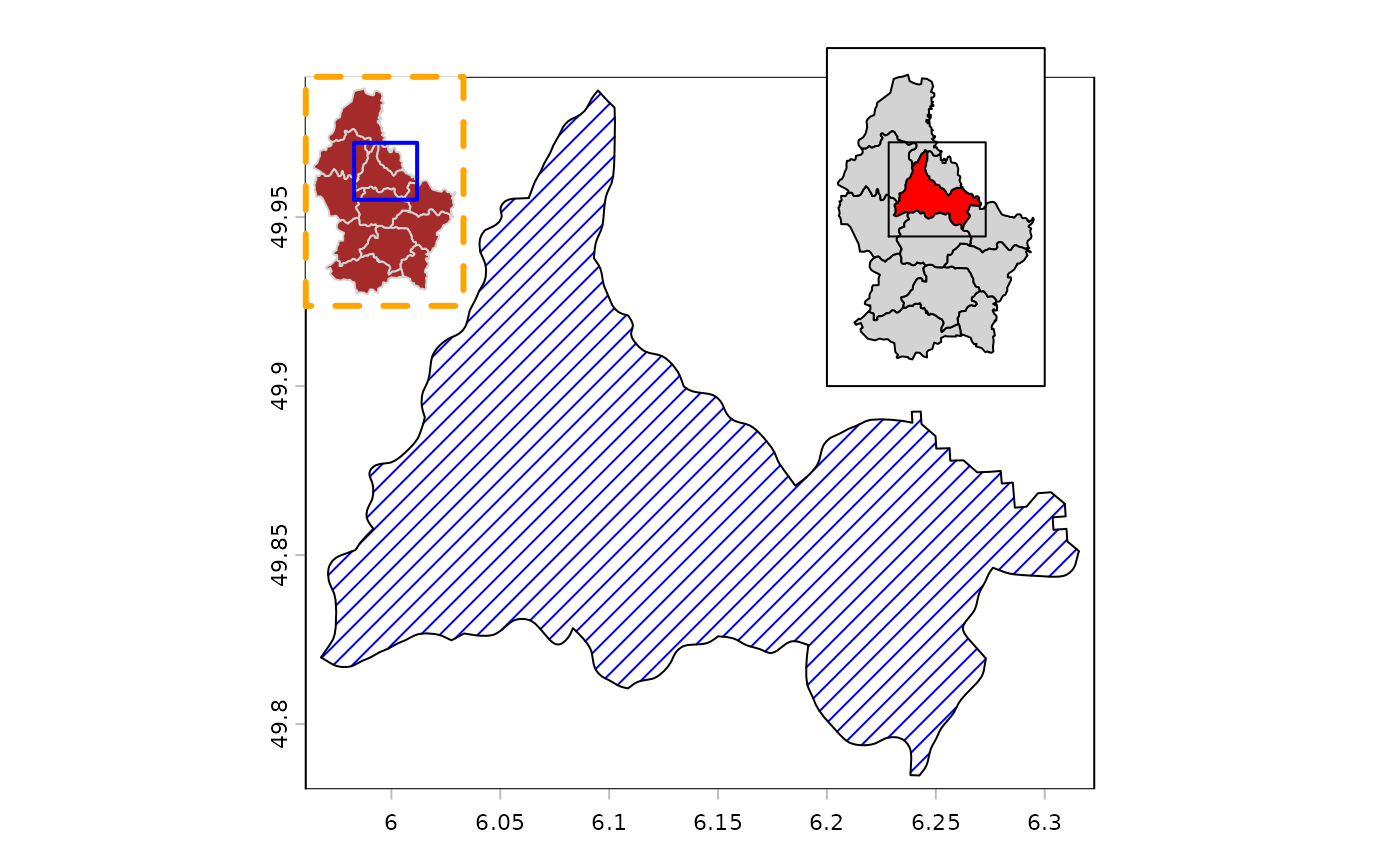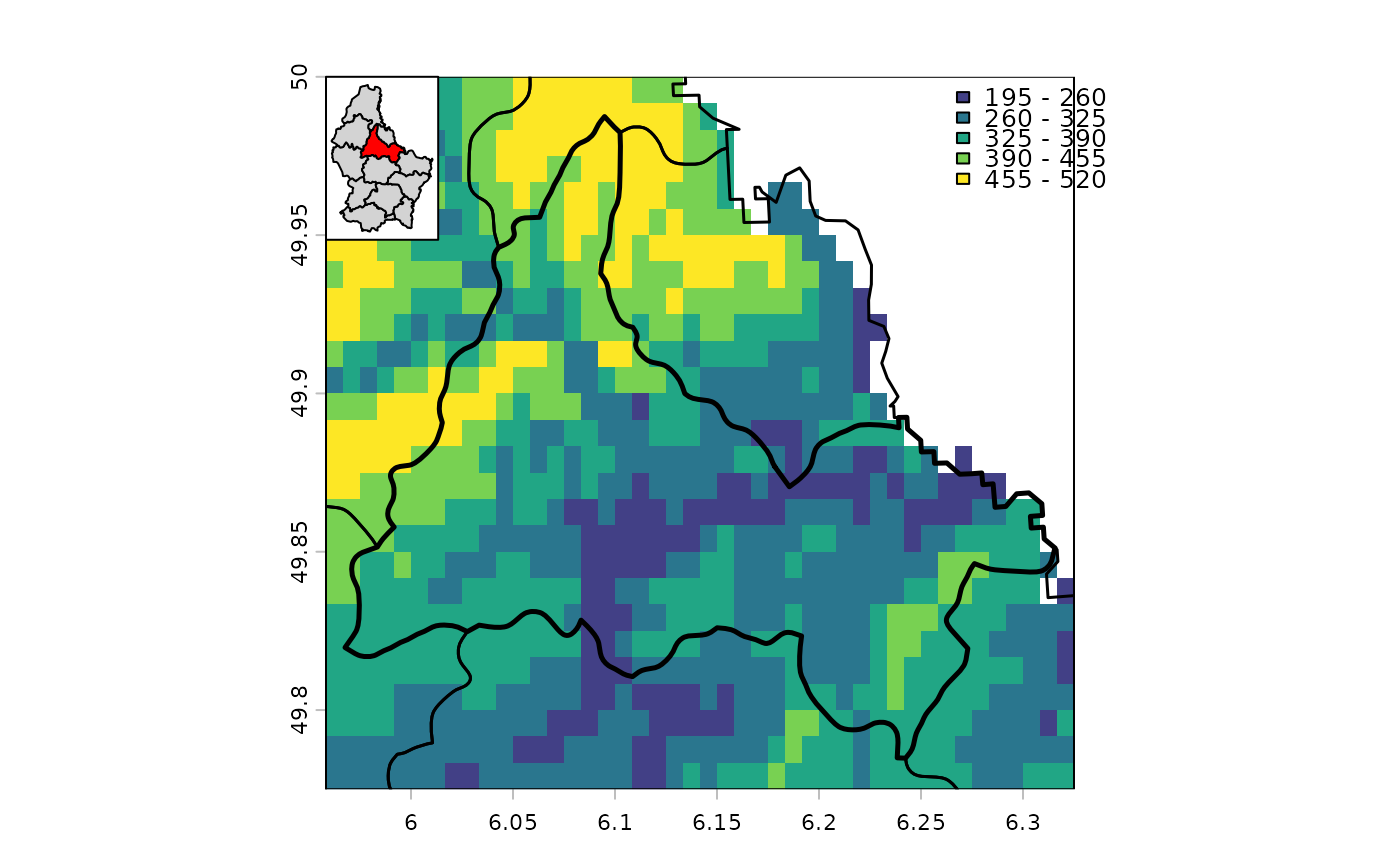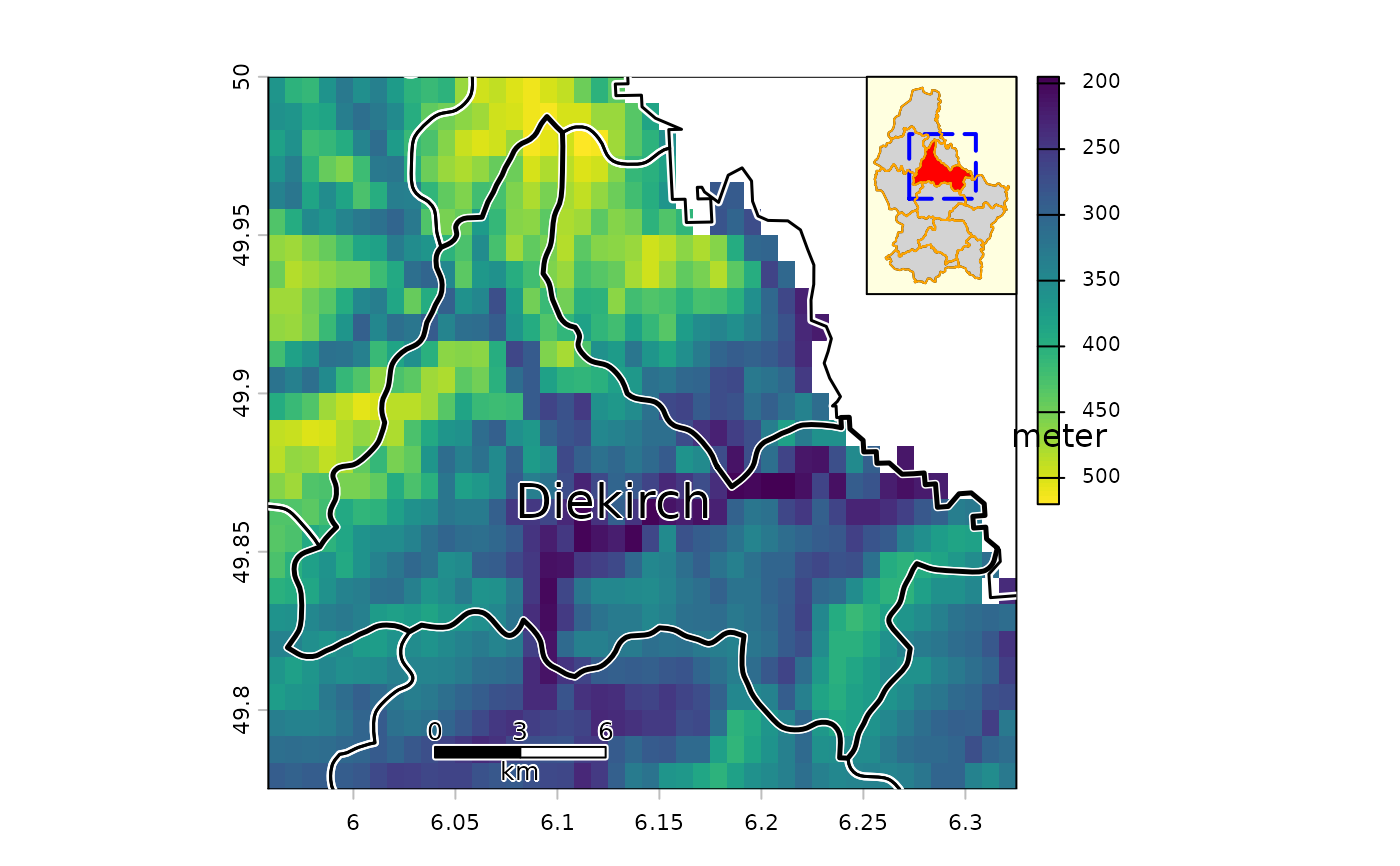Make an inset map or scale the extent of a SpatVector

# S4 method for SpatVector
inset(x, e, loc="", scale=0.2, background="white",
perimeter=TRUE, box=NULL, pper, pbox, ...)

# S4 method for SpatRaster
inset(x, e, loc="", scale=0.2, background="white",
perimeter=TRUE, box=NULL, pper, pbox, ...)

# S4 method for SpatVector
inext(x, e, y=NULL, gap=0)

## Arguments

x

SpatVector, SpatRaster

e

SpatExtent to set the size and location of the inset. Or missing

loc

character. One of "bottomright", "bottom", "bottomleft", "left", "topleft", "top", "topright", "right", "center"

scale

numeric. The relative size of the inset, used when x is missing

background

color for the background of the inset. Use NA for no background color

perimeter

logical. If TRUE a perimeter (border) is drawn around the inset

box

SpatExtent or missing, to draw a box on the inset, e.g. to show where the map is located in a larger area

pper

list with graphical parameters (arguments) such as col and lwd for the perimeter line

pbox

list with graphical parameters (arguments) such as col and lwd for the box (line)

...

additional arguments passed to plot for the drawing of x

y

SpatVector. If not NULL, y is scaled based with the parameters for x. This is useful, for example, when x represent boundaries, and y points within these boundaries

gap

numeric to add space between the SpatVector and the SpatExtent

sbar, rescale, shift

## Value

scaled and shifted SpatVector or SpatRaster (returned invisibly)

## Examples

f <- system.file("ex/lux.shp", package="terra")
v <- vect(f)
x <- v[v\$NAME_2 == "Diekirch", ]

plot(x, density=10, col="blue")
inset(v)# more elaborate
plot(x, density=10, col="blue")
inset(v, col = "brown", border="lightgrey", perimeter=TRUE,
pper=list(col="orange", lwd=3, lty=2),
box=ext(x), pbox=list(col="blue", lwd=2))

cols <- rep("light grey", 12)
cols <- "red"
e <- ext(c(6.2, 6.3, 49.9, 50))
b <- ext(x)+0.02
inset(v, e=e, col=cols, box=b)# with a SpatRaster
ff <- system.file("ex/elev.tif", package="terra")
r <- rast(ff)
r <- crop(r, ext(x) + .01)
plot(r, type="int", mar=c(2,2,2,2), plg=list(x="topright"))
lines(v, lwd=1.5)
lines(x, lwd=2.5)
inset(v, col=cols, loc="topleft", scale=0.15)# a more complex one
plot(r, plg=list(title="meter\n", shrink=.2, cex=.8))
lines(v, lwd=4, col="white")
lines(v, lwd=1.5)
lines(x, lwd=2.5)
text(x, "NAME_2", cex=1.5, halo=TRUE)
sbar(6, c(6.04, 49.785), type="bar", below="km", label=c(0,3,6), cex=.8)
s <- inset(v, col=cols, box=b, scale=.2, loc="topright", background="light yellow",
pbox=list(lwd=2, lty=5, col="blue"))

# note the returned inset SpatVector
s
#>  class       : SpatVector
#>  geometry    : polygons
#>  dimensions  : 12, 6  (geometries, attributes)
#>  extent      : 6.255333, 6.321333, 49.93245, 49.99422  (xmin, xmax, ymin, ymax)
#>  source      : lux.shp
#>  coord. ref. : lon/lat WGS 84 (EPSG:4326)
#>  names       :  ID_1   NAME_1  ID_2   NAME_2  AREA   POP
#>  type        : <num>    <chr> <num>    <chr> <num> <int>
#>  values      :     1 Diekirch     1 Clervaux   312 18081
#>                    1 Diekirch     2 Diekirch   218 32543
#>                    1 Diekirch     3  Redange   259 18664
lines(s, col="orange")